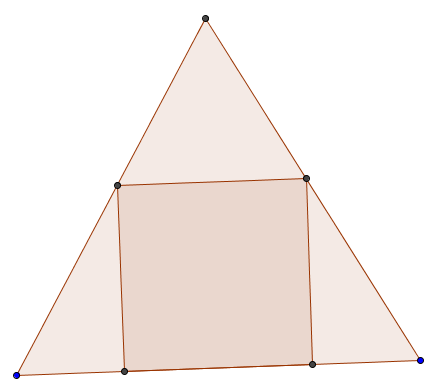# Inscribed square

Geometry Level 3A square is inscribed into an equilateral triangle as shown in the image above. If triangle's edge is $a$, calculate square's edge in terms of $a$.

×